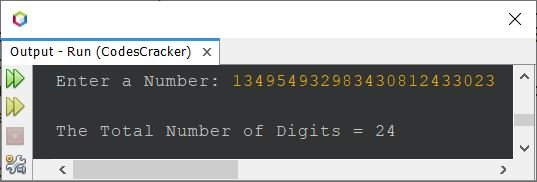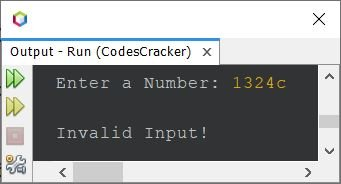# Java Program to Count Total Number of Digits in a Number

This article covers a Java program to find and print the total number of digits available in a number entered by user at run-time of the program. The program is created in multiple ways here.

## Count Total Number of Digits in Java using while Loop

The question is, write a Java program to count the number of digits available in a given number using while loop. The program given below is the answer to this question:

```import java.util.Scanner;

public class CodesCracker
{
public static void main(String[] args)
{
int num, totalDigits=0;
Scanner s = new Scanner(System.in);

System.out.print("Enter a Number: ");
num = s.nextInt();

while(num!=0)
{
totalDigits++;
num = num/10;
}

System.out.println("\nThe Total Number of Digits = " +totalDigits);
}
}```

Here is its sample run with user input 10294 as number to count all of its digits:## Count Total Number of Digits in Java using for Loop

The above program can also be created using for loop. Here is the program, counts total number of digits available in a number entered by user, using for loop, this time:

```import java.util.Scanner;

public class CodesCracker
{
public static void main(String[] args)
{
int num, totalDigits;
Scanner s = new Scanner(System.in);

System.out.print("Enter a Number: ");
num = s.nextInt();

for(totalDigits=0; num!=0; num=num/10)
totalDigits++;

System.out.println("\nThe Total Number of Digits = " +totalDigits);
}
}```

This program produces same output as of previous Java program.

Since the range of int data type in Java, is only 2,147,483,647, therefore you can change the data type from int to long whose range is 9,223,372,036,854,775,807. To do this, replace the following statement from above program:

`int num, totalDigits;`

with

`long num, totalDigits;`

And the following statement:

`num = s.nextInt();`

will get replaced with the statement given below:

`num = s.nextLong();`

That is, int to long and nextInt() to nextFloat().

But what if user enters a number who is even greater than the maximum value, a long type variable can hold. Therefore in that case, better to go with string type.

## Count Digits in a Number using String

Here is the program, finds total number of digits of a number entered by user, using string. This program does not cares about the size of number, or how big the number is.

```import java.util.Scanner;

public class CodesCracker
{
public static void main(String[] args)
{
String num;
int totalDigits;
Scanner s = new Scanner(System.in);

System.out.print("Enter a Number: ");
num = s.next();

totalDigits = num.length();

System.out.println("\nThe Total Number of Digits = " +totalDigits);
}
}```

Here is its sample run with user input 134954932983430812433023:Now the question may arise, what if user enters string in place of integer ?
Then in that case, you can check whether each and every character of given string is a digit or not. Here is the program, that provides the complete solution I think, of counting digits of an integer in Java:

```import java.util.Scanner;

public class CodesCracker
{
public static void main(String[] args)
{
String num;
int totalDigits;
char ch;
Scanner s = new Scanner(System.in);

System.out.print("Enter a Number: ");
num = s.next();

totalDigits = num.length();
for(int i=0; i<totalDigits; i++)
{
ch = num.charAt(i);
if(!Character.isDigit(ch))
{
System.out.println("\nInvalid Input!");
return;
}
}

System.out.println("\nThe Total Number of Digits = " +totalDigits);
}
}```

Here is its sample run with user input 1324c:Java Online Test

« Previous Program Next Program »